# Collinearity (Reading: Faraway (2005, 1st edition), 5.3)

The Longley dataset used in previous lab is a good example of collinearity:

> data(longley)

> g <- lm(Employed ~ ., data=longley)
> summary(g)

Coefficients:

Estimate Std. Error t value Pr(>|t|)

(Intercept)  -3.482e+03  8.904e+02  -3.911 0.003560 **

GNP.deflator  1.506e-02  8.492e-02   0.177 0.863141

GNP          -3.582e-02  3.349e-02  -1.070 0.312681

Unemployed   -2.020e-02  4.884e-03  -4.136 0.002535 **

Armed.Forces -1.033e-02  2.143e-03  -4.822 0.000944 ***

Population   -5.110e-02  2.261e-01  -0.226 0.826212

Year          1.829e+00  4.555e-01   4.016 0.003037 **

---

Signif. codes:  0 `***' 0.001 `**' 0.01 `*' 0.05 `.' 0.1 ` ' 1

Residual standard error: 0.3049 on 9 degrees of freedom

Multiple R-Squared: 0.9955,     Adjusted R-squared: 0.9925

F-statistic: 330.3 on 6 and 9 DF,  p-value: 4.984e-10

Recall that the response is number employed. Three of the predictors have large p-values but all are variables that might be expected to affect the response. Why aren't they significant? Check the correlation matrix first (rounding to 3 digits for convenience)

> round(cor(longley[,-7]),3)

GNP.deflator   GNP Unemployed Armed.Forces Population  Year

GNP.deflator        1.000 0.992      0.621        0.465      0.979 0.991

GNP                 0.992 1.000      0.604        0.446      0.991 0.995

Unemployed          0.621 0.604      1.000       -0.177      0.687 0.668

Armed.Forces        0.465 0.446     -0.177        1.000      0.364 0.417

Population          0.979 0.991      0.687        0.364      1.000 0.994

Year                0.991 0.995      0.668        0.417      0.994 1.000

There are several large pairwise correlations. It can be also revealed from their pairwise scatter plots:

> x <- as.matrix(longley[,-7])

> pairs(x)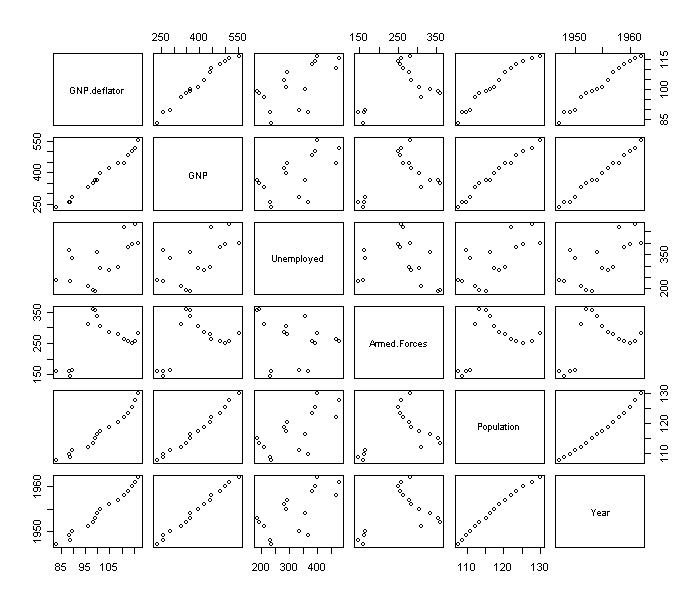Now we check the eigen-decomposition:

> e <- eigen( t(x) %*% x)
> e

\$values

 6.665299e+07 2.090730e+05 1.053550e+05 1.803976e+04 2.455730e+01 2.015117e+00

\$vectors

[,1]        [,2]        [,3]        [,4]        [,5]          [,6]

[1,] -0.04990131  0.06979071 -0.03416853  0.04265870  0.95653127 -0.2733126381

[2,] -0.19075418  0.72496814 -0.34330489  0.55402997 -0.07487553  0.0872940138

[3,] -0.15702286  0.62152746  0.56371985 -0.52067703 -0.00716578  0.0105568115

[4,] -0.12796016  0.10434859 -0.74630465 -0.64468394 -0.01222896 -0.0001122542

[5,] -0.05758090  0.03841364 -0.01095845  0.03583083 -0.28108541 -0.9564496276

[6,] -0.95748481 -0.26625145  0.07812474  0.05679111 -0.01522131  0.0526555591

> sqrt(e\$value/e\$value)
    1.00000   17.85504   25.15256   60.78472 1647.47771 5751.21560

There is a wide range in the eigenvalues and several condition numbers are large. This means that problems are being caused by more than just one linear combination. Now check out the variance inflation factors (VIF). For the first variable this is:

> summary(lm( x[,1] ~ x[,-1]))\$r.squared
 0.9926217

> 1/(1-0.9926217)
 135.5326

which is large - the VIF for orthogonal predictors is 1. Here is a function to compute all the VIF's in one go:

> vif(x)
  135.53244 1788.51348   33.61889    3.58893  399.15102  758.98060

There's definitely a lot of variance inflation! For example, we can interpret sqrt(1788)=42.28 as telling us that the standard error for GNP is 42 times larger than it would have been without collinearity. How can we get rid of this problem? One way is to throw out some of the variables. Examine the full correlation matrix above. Notice that variables 3 and 4 (Unemployed and Armed.Forces) do not have extremely large pairwise correlations with the other variables so we should keep them and focus on the others for candidates for removal:

> cor(x[,-c(3,4)])
GNP deflator       GNP Population      Year
GNP deflator    1.0000001 0.9915892  0.9791635 0.9911493
GNP    0.9915892 1.0000000  0.9910901 0.9952735
Population    0.9791635 0.9910901  1.0000001 0.9939529
Year    0.9911493 0.9952735  0.9939529 1.0000000

These four variables are strongly correlated with each other - any one of them could do the job of representing the others - we could pick year arbitrarily:

> summary(lm(Employed ~ Armed.Forces + Unemployed + Year, data=longley))

Coefficients:

Estimate Std. Error t value Pr(>|t|)

(Intercept)  -1.797e+03  6.864e+01 -26.183 5.89e-12 ***

Armed.Forces -7.723e-03  1.837e-03  -4.204  0.00122 **

Unemployed   -1.470e-02  1.671e-03  -8.793 1.41e-06 ***

Year          9.564e-01  3.553e-02  26.921 4.24e-12 ***

---

Signif. codes:  0 `***' 0.001 `**' 0.01 `*' 0.05 `.' 0.1 ` ' 1

Residual standard error: 0.3321 on 12 degrees of freedom

Multiple R-Squared: 0.9928,     Adjusted R-squared: 0.9911

F-statistic: 555.2 on 3 and 12 DF,  p-value: 3.916e-13

Compare this with the original fit - what do you find? We see that the fit is very similar but only three rather than six predictors are used.

One final point - extreme collinearity can cause problems in computing the estimates - look what happens when we use the direct formula for b-hat on the data with a 100 times larger scale change on first predictor:

> xsc <- as.matrix(cbind(1, 100*x[,1], x[,2:6]))
> solve(t(xsc) %*% xsc) %*% t(xsc) %*% longley\$Employed
Error in solve.default(t(xsc) %*% xsc) : singular matrix `a' in solve

R, like most statistical packages, uses a more numerically stable method for computing the estimates in lm().

> lm(longley\$Employed~xsc-1)

Call:

lm(formula = longley\$Employed ~ xsc - 1)

Coefficients:

xsc              xsc           xscGNP    xscUnemployed  xscArmed.Forces

-3.482e+03        1.506e-04       -3.582e-02       -2.020e-02       -1.033e-02

xscPopulation          xscYear

-5.110e-02        1.829e+00

# Principal Components (Reading: Faraway (2005, 1st edition), 9.1)

We use the Longley data for this example:

> data(longley)

> longley # take a look

> x <- as.matrix(longley[,-7])

> y <- longley[,7]

First we compute the eigen-decomposition for XTX
> e <- eigen(t(x) %*% x)

Look at the eigenvalues:

> e\$values
 6.665299e+07 2.090730e+05 1.053550e+05 1.803976e+04 2.455730e+01 2.015117e+00

Look at the relative size - the first is big. Consider the first eigenvector (column) below:

> dimnames(e\$vectors) <- list(c("GNP def","GNP","Unem","Armed","Popn","Year"),paste("EV",1:6))

> e\$vectors

EV 1        EV 2        EV 3        EV 4        EV 5          EV 6

GNP def -0.04990131  0.06979071 -0.03416853  0.04265870  0.95653127 -0.2733126381

GNP     -0.19075418  0.72496814 -0.34330489  0.55402997 -0.07487553  0.0872940138

Unem    -0.15702286  0.62152746  0.56371985 -0.52067703 -0.00716578  0.0105568115

Armed   -0.12796016  0.10434859 -0.74630465 -0.64468394 -0.01222896 -0.0001122542

Popn    -0.05758090  0.03841364 -0.01095845  0.03583083 -0.28108541 -0.9564496276

Year    -0.95748481 -0.26625145  0.07812474  0.05679111 -0.01522131  0.0526555591

The first eigenvector is dominated by Year (because the coefficient for Year in the 1st eigenvector is 0.95748481, which is much larger than other coefficients). Why is it? Now examining the X-matrix. What are the scales of the variables?

> x
GNP deflator     GNP Unemployed Armed Forces Population Year
1947         83.0 234.289      235.6        159.0    107.608 1947
1948         88.5 259.426      232.5        145.6    108.632 1948
1949         88.2 258.054      368.2        161.6    109.773 1949
1950         89.5 284.599      335.1        165.0    110.929 1950
1951         96.2 328.975      209.9        309.9    112.075 1951
1952         98.1 346.999      193.2        359.4    113.270 1952
1953         99.0 365.385      187.0        354.7    115.094 1953
1954        100.0 363.112      357.8        335.0    116.219 1954
1955        101.2 397.469      290.4        304.8    117.388 1955
1956        104.6 419.180      282.2        285.7    118.734 1956
1957        108.4 442.769      293.6        279.8    120.445 1957
1958        110.8 444.546      468.1        263.7    121.950 1958
1959        112.6 482.704      381.3        255.2    123.366 1959
1960        114.2 502.601      393.1        251.4    125.368 1960
1961        115.7 518.173      480.6        257.2    127.852 1961
1962        116.9 554.894      400.7        282.7    130.081 1962

We see that these predictors have values in very different regions. The reason that Year stands up in the 1st principal component is because its values are much larger than others. It might make more sense to center the predictors before trying principal components. This is equivalent to doing principal components on the covariance matrix:

> e <- eigen(cov(x))

> e\$values

 1.535852e+04 7.077406e+03 1.204409e+03 1.638766e+00 1.385096e-01 2.836721e-02

> plot(e\$values )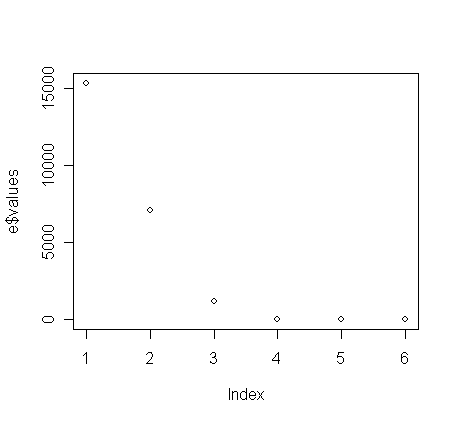> dimnames(e\$vectors) <- list(c("GNP def","GNP","Unem","Armed","Popn","Year"),paste("EV",1:6))

> e\$vectors

EV 1        EV 2        EV 3        EV 4         EV 5         EV 6

GNP def -0.08247788  0.03437017  0.04184609  0.95671439 -0.269588027  0.047800192

GNP     -0.75623528  0.31936977  0.55729097 -0.07632282  0.076772448 -0.061795997

Unem    -0.62622375 -0.58026266 -0.52050055 -0.00739462  0.008954116 -0.009131889

Armed   -0.15750866  0.74819438 -0.64438951 -0.01231829 -0.000605424 -0.002500043

Popn    -0.05439163  0.01348608  0.03425705 -0.27999345 -0.927886716  0.237310015

Year    -0.03717521  0.01184015  0.01853299  0.01642086  0.245711186  0.968241040

Can you give these P.C.'s (at least the first 3 P.C's) an interpretation based on the values in their eigenvectors? It can be observed that now GNP, Unemployed, and Armed Forces play the most important roles in the first three principal components, whose corresponding eigenvalues are large in contrast to rest eigenvalues. Why is it? Let's check the variances of these variables (see below). What have you observed and can you now understand why it happens?

> var(x)

GNP.deflator       GNP Unemployed Armed.Forces Population      Year

GNP.deflator    116.45762 1063.6041   625.8666     349.0254   73.50300  50.92333

GNP            1063.60412 9879.3537  5612.4370    3088.0428  685.24094 470.97790

Unemployed      625.86663 5612.4370  8732.2343   -1153.7876  446.27415 297.30333

Armed.Forces    349.02538 3088.0428 -1153.7876    4843.0410  176.40981 138.24333

Population       73.50300  685.2409   446.2742     176.4098   48.38735  32.91740

Year             50.92333  470.9779   297.3033     138.2433   32.91740  22.66667

You can examine if Si var(xi)  equals sum of eigenvalues.

> sum(diag(var(x)))

 23642.14

> sum(e\$values)

 23642.14

Notice that the two values are identical. Why?

We can create the orthogonalized predictors --- the Z=XU operation as follows:

> xpc <- x %*% e\$vector
> round(cov(xpc),3)

EV 1     EV 2     EV 3  EV 4  EV 5  EV 6

EV 1 15358.52    0.000    0.000 0.000 0.000 0.000

EV 2     0.00 7077.406    0.000 0.000 0.000 0.000

EV 3     0.00    0.000 1204.409 0.000 0.000 0.000

EV 4     0.00    0.000    0.000 1.639 0.000 0.000

EV 5     0.00    0.000    0.000 0.000 0.139 0.000

EV 6     0.00    0.000    0.000 0.000 0.000 0.028

We can see that these new predictors, i.e. the principal components, are mutually orthogonal, and please note their variances are equal to their corresponding eigenvalues. Because these P.C.'s are obtained from non-centered X-matrix, they are not orthogonal to the constant term. You can check it by:

> round(summary(lm(y~xpc),cor=T)\$cor,3)

To generate centered X-matrix,  you can use:

> xmean <- apply(x, 2, mean) # apply mean operation on the columns of x
> cx <- sweep(x, 2, xmean) # sweep out mean on each columns of x

or equivalently use the command "cx <- scale(x, center=T, scale=F)".

We now create the orthogonalized predictors using centered X-matrix:
> cxpc <- cx %*% e\$vector

Try to verify if the new predictors in cxpc are mutually orthogonal and orthogonal to the constant term in regression analysis:

> summary(lm(y~cxpc),cor=T)

Call:

lm(formula = y ~ xpc)

Residuals:

Min       1Q   Median       3Q      Max

-0.41011 -0.15767 -0.02816  0.10155  0.45539

Coefficients:

Estimate Std. Error t value Pr(>|t|)

(Intercept) -3.482e+03  8.904e+02  -3.911  0.00356 **

xpcEV 1     -2.510e-02  6.351e-04 -39.511 2.12e-11 ***

xpcEV 2      1.404e-02  9.356e-04  15.004 1.13e-07 ***

xpcEV 3      2.999e-02  2.268e-03  13.223 3.36e-07 ***

xpcEV 4      6.177e-02  6.149e-02   1.005  0.34137

xpcEV 5      4.899e-01  2.115e-01   2.316  0.04577 *

xpcEV 6      1.762e+00  4.673e-01   3.770  0.00441 **

---

Signif. codes:  0 '***' 0.001 '**' 0.01 '*' 0.05 '.' 0.1 ' ' 1

Residual standard error: 0.3049 on 9 degrees of freedom

Multiple R-Squared: 0.9955,     Adjusted R-squared: 0.9925

F-statistic: 330.3 on 6 and 9 DF,  p-value: 4.984e-10

Correlation of Coefficients:

(Intercept) xpcEV 1 xpcEV 2 xpcEV 3 xpcEV 4 xpcEV 5

xpcEV 1  0.00

xpcEV 2  0.00        0.00

xpcEV 3  0.00        0.00    0.00

xpcEV 4  0.00        0.00    0.00    0.00

xpcEV 5 -0.09        0.00    0.00    0.00    0.00

xpcEV 6 -1.00        0.00    0.00    0.00    0.00    0.00

In the fitted model, the b estimate, interpretation, and t-tests are much simplified because of the orthogonality between predictors.

Suppose you feel that the P.C.'s should not be influenced by the means and variances of the predictors. You may like to normalize these predictors to a same scale before trying principal component. This is equivalent to apply eigen-decomposition on its correlation matrix:

> e <- eigen(cor(x))

> e\$values

 4.6033770958 1.1753404993 0.2034253724 0.0149282587 0.0025520658 0.0003767081

> sum(e\$values)

 6
Now, sum of eigenvalues = 6, which equals the number of predictors, why?

> dimnames(e\$vectors) <- list(c("GNP def","GNP","Unem","Armed","Popn","Year"),paste("EV",1:6))

> e\$vectors

EV 1          EV 2       EV 3         EV 4         EV 5        EV 6

GNP def 0.4618349  0.0578427677  0.1491199  0.792873559 -0.337937826  0.13518707

GNP     0.4615043  0.0532122862  0.2776823 -0.121621225  0.149573192 -0.81848082

Unem    0.3213167 -0.5955137627 -0.7283057  0.007645795 -0.009231961 -0.10745268

Armed   0.2015097  0.7981925480 -0.5616075 -0.077254979 -0.024252472 -0.01797096

Popn    0.4622794 -0.0455444698  0.1959846 -0.589744965 -0.548578173  0.31157087

Year    0.4649403  0.0006187884  0.1281157 -0.052286554  0.749542836  0.45040888

Can you give these P.C.'s (at least the first 3 P.C's) an interpretation based on the values in their eigenvectors?

One commonly used method of judging how many principal components are worth considering is to plot eigenvalues:

> plot(e\$values, type="l", xlab="EV no.")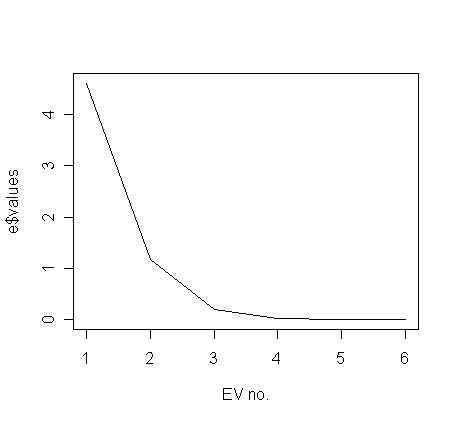Often, the plot has a noticeable "elbow". Here the elbow is at about 3, which tells us that we need only consider the first two principle components. The first 2 P.C.'s explain about 94.9% (=0.650+0.299, see below) variation in the normalized X-matrix.

> round(e\$value/sum(e\$value),3)
 0.650 0.299 0.051 0.000 0.000 0.000

One advantage of principal components is that it transforms the predictors to an orthogonal basis. To figure out the orthogonalized predictors for this data based on the eigen-decomposition for the correlation matrix of X, we must first normalize the data: The functions "scale()" is useful for doing this:

> nx <- scale(x) # the default is "center=Ture and scale=Ture"

Let us take a look of the covariance matrix of nx to examine whether it is normalized:

> cov(nx)

GNP.deflator       GNP Unemployed Armed.Forces Population      Year

GNP.deflator    1.0000000 0.9915892  0.6206334    0.4647442  0.9791634 0.9911492

GNP             0.9915892 1.0000000  0.6042609    0.4464368  0.9910901 0.9952735

Unemployed      0.6206334 0.6042609  1.0000000   -0.1774206  0.6865515 0.6682566

Armed.Forces    0.4647442 0.4464368 -0.1774206    1.0000000  0.3644163 0.4172451

Population      0.9791634 0.9910901  0.6865515    0.3644163  1.0000000 0.9939528

Year            0.9911492 0.9952735  0.6682566    0.4172451  0.9939528 1.0000000

We can now create the orthogonalized predictors, i.e., the P.C.'s, by;

> nxpc <- nx %*% e\$vector

and fit:

> g <- lm(y~nxpc)

> summary(g)

Coefficients:

Estimate Std. Error t value Pr(>|t|)

(Intercept) 65.31700    0.07621 857.026  < 2e-16 ***

nxpcEV 1     1.56511    0.03669  42.662 1.07e-11 ***

nxpcEV 2     0.39183    0.07260   5.397 0.000435 ***

nxpcEV 3    -1.86039    0.17452 -10.660 2.10e-06 ***

nxpcEV 4     0.35730    0.64423   0.555 0.592672

nxpcEV 5    -6.16983    1.55812  -3.960 0.003305 **

nxpcEV 6     6.96337    4.05550   1.717 0.120105

---

Signif. codes:  0 `***' 0.001 `**' 0.01 `*' 0.05 `.' 0.1 ` ' 1

Residual standard error: 0.3049 on 9 degrees of freedom

Multiple R-Squared: 0.9955,     Adjusted R-squared: 0.9925

F-statistic: 330.3 on 6 and 9 DF,  p-value: 4.984e-10

Notice that the p-values of the 4th and 6th P.C.'s are not significant while the 5th is. Because the directions of the eigenvectors are set successively in the greatest remaining direction of variation in the predictors, it's natural to expect that they are ordered in significance in predicting the response. However, there is no guarantee of this --- we see here that the 5th P.C. is significant while the 4th is not even though there is about six times greater variation in the 4th direction than the 5th. In this example, it hardly matters since most of the variation is explained by the earlier values, but look out for this effect in other dataset in the first few eigenvalues.

Check if all the predictors in model g are orthogonal:

> round(summary(g,cor=T)\$cor,3)

(Intercept) nxpcEV 1 nxpcEV 2 nxpcEV 3 nxpcEV 4 nxpcEV 5 nxpcEV 6

(Intercept)           1        0        0        0        0        0        0

nxpcEV 1              0        1        0        0        0        0        0

nxpcEV 2              0        0        1        0        0        0        0

nxpcEV 3              0        0        0        1        0        0        0

nxpcEV 4              0        0        0        0        1        0        0

nxpcEV 5              0        0        0        0        0        1        0

nxpcEV 6              0        0        0        0        0        0        1

Notice now the P.C.'s are not only mutually orthogonal, they are also orthogonal to the constant term.

Principal components are really only useful if we can interpret the meaning of the new linear combinations. Look back at the first eigenvector - this is roughly a linear combination of all the (normalized variables). Now plot each of the variables as they change over time:
> par(mfrow=c(2,3))
> for(i in 1:6) plot(longley[,6], longley[,i], type="l", xlab="Year", ylab=names(longley)[i])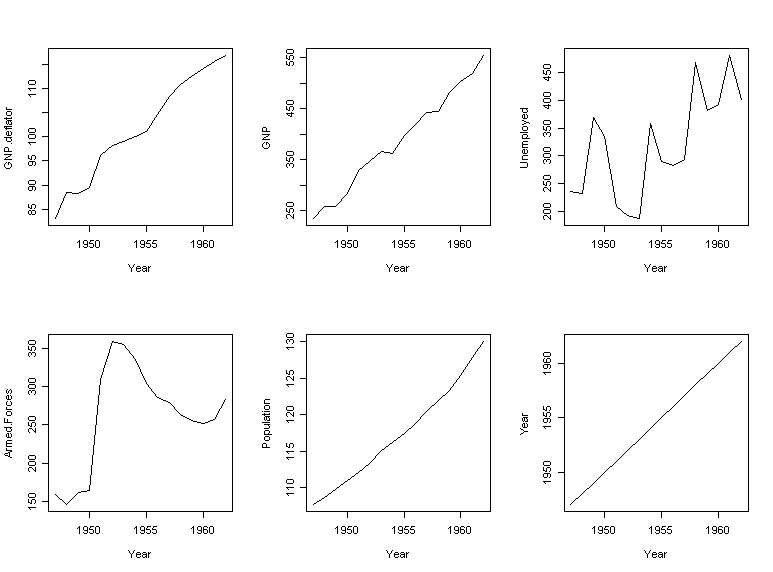What do you notice? This suggests we identify the first principal component with a time trend effect. The second principal component is roughly a contrast between numbers unemployed and in the armed forces. Let's try fitting a regression with those two components:

> summary(lm(Employed ~ Year + I(Armed.Forces-Unemployed), data=longley))

Coefficients:

Estimate Std. Error t value Pr(>|t|)

(Intercept)                  -1.391e+03  7.889e+01 -17.638 1.84e-10 ***

Year                          7.454e-01  4.037e-02  18.463 1.04e-10 ***

I(Armed.Forces - Unemployed)  4.119e-03  1.525e-03   2.701   0.0182 *

---

Signif. codes:  0 `***' 0.001 `**' 0.01 `*' 0.05 `.' 0.1 ` ' 1

Residual standard error: 0.7178 on 13 degrees of freedom

Multiple R-Squared: 0.9638,     Adjusted R-squared: 0.9582

F-statistic:   173 on 2 and 13 DF,  p-value: 4.285e-10

This approaches the fit of the full model and is easily interpretable. We need to do more work on the other principal components.

This example illustrates a typical use of principal components for regression. Some intuition is required to form new variables as combinations of older ones. It it works, a simplified and interpretable model is obtained, but it doesn't always work out that way.

# Ridge regression (Reading: Faraway (2005, 1st edition), 9.3)

We demonstrate the method on Longley data. You will need to load a library called "MASS" to help you on the analysis:

> data(longley)

> library(MASS)

> gr <- lm.ridge(Employed~., longley, lambda=seq(0,0.1,by=0.001)) # explore ridge trace in the region lÎ[0, 0.1]

Let's plot the ridge trace:

> matplot(gr\$lambda, t(gr\$coef), type="l", xlab=expression(lambda), ylab=expression(hat(gamma)), lwd=3)
> abline(h=0, lwd=3)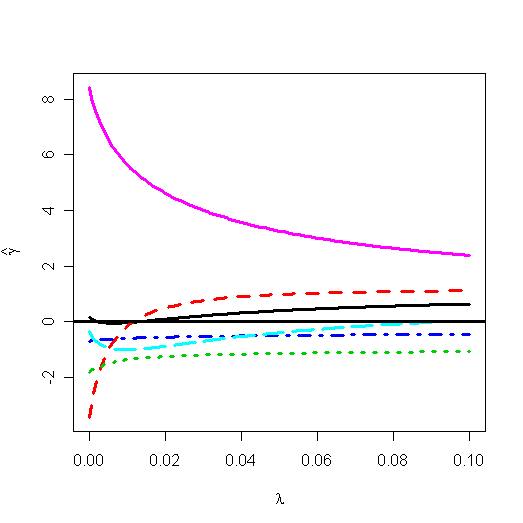You can find the ridge trace plot in graphic window. There are some automate methods to select an appropriate l:
> select(gr)
modified HKB estimator is 0.004275357
modified L-W estimator is 0.03229531
smallest value of GCV at 0
> abline(v=0.004275357, lty=10, lwd=3) # the HKB estimator
> abline(v=0.03229531, lty=11, lwd=3) # the L-W estimator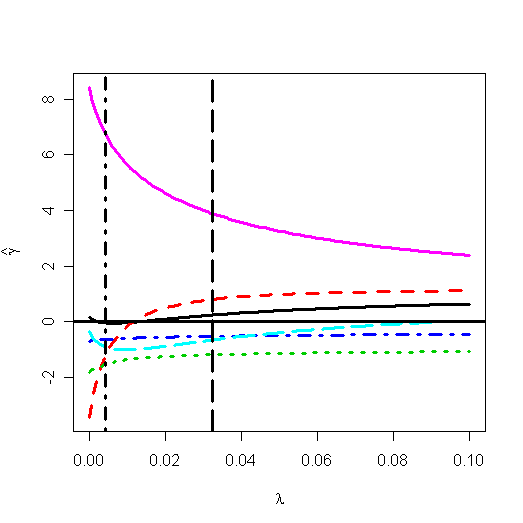The Hoerl-Kennard (the originators of ridge regression) choice and L-W choice of l has been shown on the plot. I would prefer the value of 0.03. For this choice of l, the estimate of b is:

> gr\$coef[, gr\$lam == 0.03]

GNP.deflator         GNP   Unemployed Armed.Forces   Population        Year
0.2200496   0.7693585   -1.1894102   -0.5223393   -0.6861816   4.0064269

In contrast to the least squares estimates:

> gr\$coef[, 1]

GNP.deflator          GNP   Unemployed Armed.Forces   Population        Year
0.1573796   -3.4471925   -1.8278860   -0.6962102   -0.3441972   8.4319716
Note that these values are based on centered and scaled predictors which explains the difference from previous fit. Consider the change in the coefficient for GNP. For the least squares fit, the effect of GNP is negative on the response --- number of people employed. This is counter-intuitive since we would expect the effect to be positive. The ridge estimate is positive, which is more in line with what we'd expect.